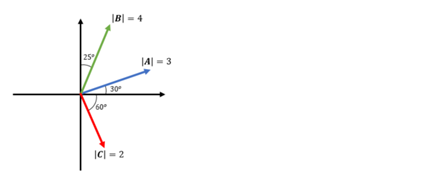# Problem: Given the vectors in the following graph, write  (A - B) + C in vector notation ( i.e  a = axî + ayĵ)

###### FREE Expert Solution
84% (443 ratings)
###### Problem Details

Given the vectors in the following graph, write  (A - B) + C in vector notation ( i.e  a = axî + ayĵ)